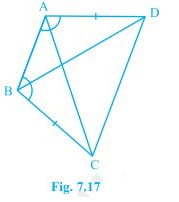# ABCD is a quadrilateral in which AD = BC and DAB = CBA (see Fig.) . Prove that (i) ΔABD ΔBAC (ii) BD = AC (iii) ABD = BACSolution

Given

AD = BC and ∠ DAB = ∠ CBA

To Prove

(i) ΔABD ΔBAC

(ii) BD = AC

(iii) ABD = BAC.

Proof

And ∠ DAB = ∠ CBA.

On onsidering two triangles ΔABD and ΔBAC.

AD = BC { As given }………………………………………….. (i)

∠ DAB = ∠ CBA { As given also}……………………….. (ii)

AB {Common side of both the triangle)…………. (iii)

From the above three equation, two triangles ABD and BAC satisfies the SAS congruency criterion

So, ΔABD ≅ ΔBAC

(ii)

BD and AC will be equal as they are corresponding parts of congruent triangles(CPCT).

So BD = AC

(iii) Similarly,

∠ABD and ∠BAC will be equal as they are corresponding parts of congruent triangles(CPCT).

So,

∠ABD = ∠BAC.